Select Page

# CBSE MCQ Maths 12 Science Three Dimensional Geometry Solutions in English

CBSE MCQ Maths 12 Science Three Dimensional Geometry Solutions in English to enable students to get Solutions in a narrative video format for the specific question.

Expert Teacher provides CBSE MCQ Maths 12 Science Three Dimensional Geometry Solutions through Video Solutions in English language. This video solution will be useful for students to understand how to write an answer in exam in order to score more marks. This teacher uses a narrative style for a question from Three Dimensional Geometry not only to explain the proper method of answering question, but deriving right answer too.

Please find the question below and view the Solution in a narrative video format.

Question:

Solution Video in English:

## Similar Questions from CBSE, 12th Science, Maths, Three Dimensional Geometry

Question 1 : The equation of a line AB is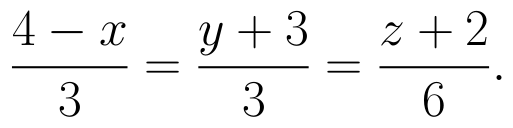Write the direction cosines of a line parallel to the above line. (View Answer Video)

Question 2 : Find the equation of the plane passing through the point (-1, 3, 2) and perpendicular to each of the planes x + 2y + 3z = 5 and 3x + 3y + z = 0. (View Answer Video)

Question 3 : Find the distance of point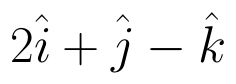from the  plane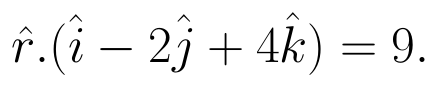(View Answer Video)

Question 4 : If a line makes angle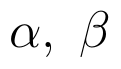and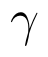with the coordinate axis, then find the value of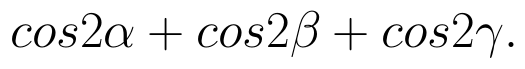(View Answer Video)

Question 5 : Find the vector equation of the line passing through the point (1, 2, 3) and parallel to the planes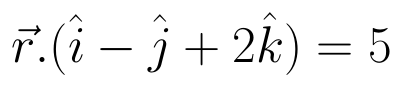and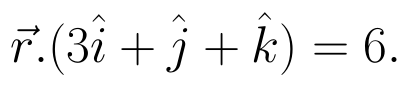(View Answer Video)

### Application of Integrals

Question 1 : Using the method of integration find the area of the region bounded by lines:
2x + y = 4, 3x - 2y = 6 and x - 3y + 5 = 0.

Question 2 : Find the area of the smaller region bounded by the ellipse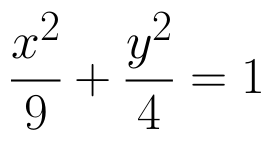and the line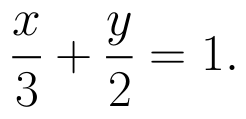(View Answer Video)

Question 3 : Find the area enclosed between the parabola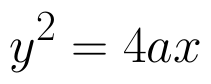and the line y = mx. (View Answer Video)

Question 4 : Using integration, find the area of the region bounded by the line 2x + y = 4, 3x - 2y = 6 and x - 3y + 5 = 0. (View Answer Video)

Question 5 : Find the area between the curves y = x and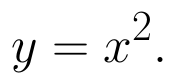(View Answer Video)

### Determinant

Question 1 : If the area of a triangle is 35 sq units with vertices (2, -6), (5, 4) and (k, 4). Then k is: (View Answer Video)

Question 2 : If A is a symmetric matrix, then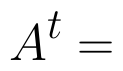? (View Answer Video)

Question 3 : Evaluate the determinant: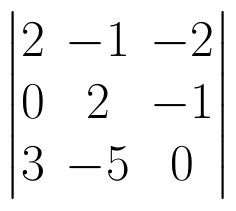. (View Answer Video)

Question 4 : If A is an invertible matrix of order 2, then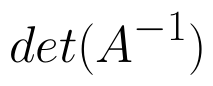is equal to, (View Answer Video)

Question 5 : Let A be the non- singular square matrix of order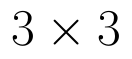, then |adj A| is equal to, (View Answer Video)

### Integrals

Question 1 : Find the integral of the function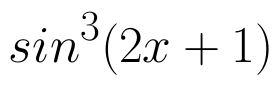. (View Answer Video)

Question 2 : Evaluate :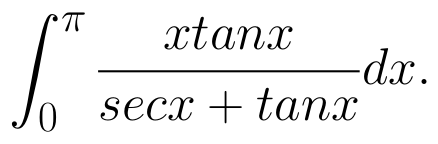(View Answer Video)

Question 3 : Find the integral of the function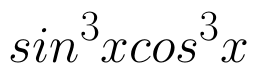. (View Answer Video)

Question 4 :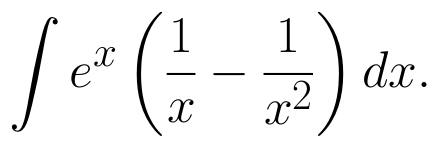(View Answer Video)

Question 5 : Evaluate :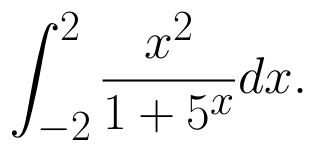(View Answer Video)